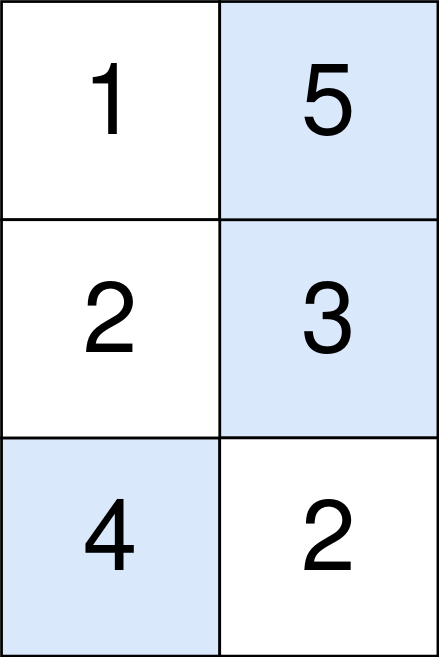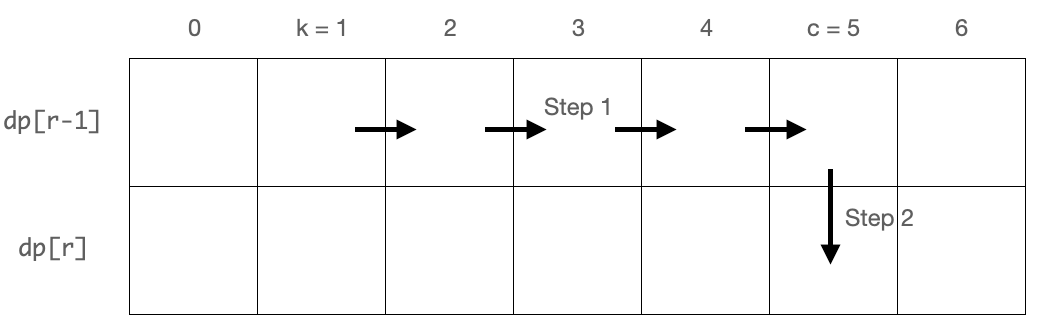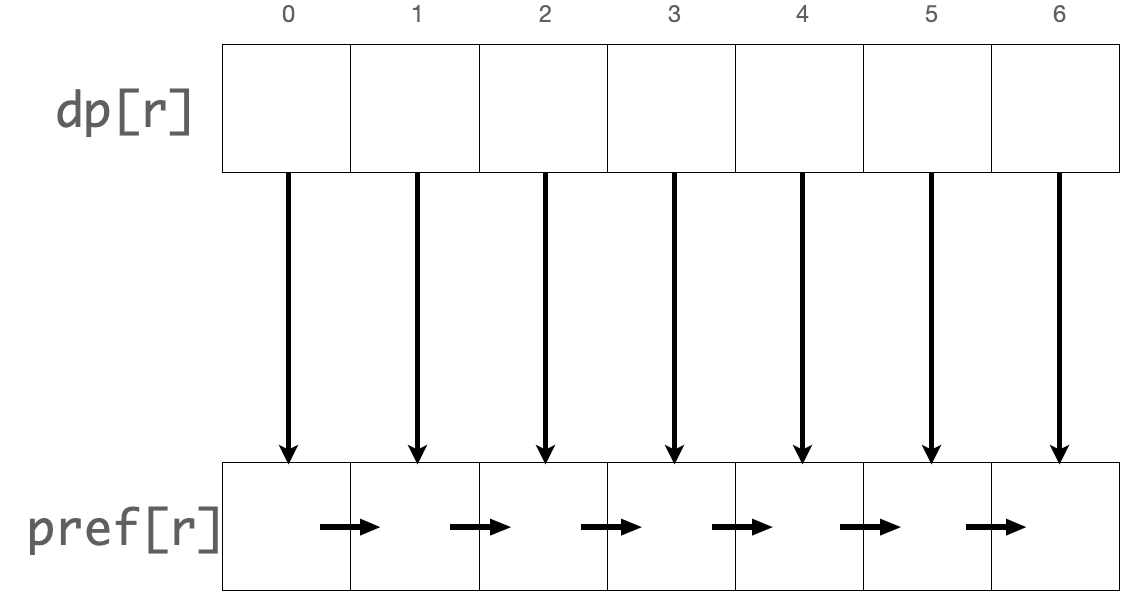# LeetCode 1937. Maximum Number of Points with Cost Solution

You are given an m x n integer matrix points (0-indexed). Starting with $0$ points, you want to maximize the number of points you can get from the matrix.

To gain points, you must pick one cell in each row. Picking the cell at coordinates (r, c) will add points[r][c] to your score.

However, you will lose points if you pick a cell too far from the cell that you picked in the previous row. For every two adjacent rows r and r + 1 (where 0 <= r < m - 1), picking cells at coordinates (r, c1) and (r + 1, c2) will subtract abs(c1 - c2) from your score.

Return the maximum number of points you can achieve.

abs(x) is defined as:

• x for x >= 0
• -x for x < 0

Example 1:Input: points = [[1,2,3],[1,5,1],[3,1,1]]
Output: $9$
Explanation:
The blue cells denote the optimal cells to pick, which have coordinates (0, 2), (1, 1), and (2, 0).
You add 3 + 5 + 3 = 11 to your score.
However, you must subtract abs(2 - 1) + abs(1 - 0) = 2 from your score.
Your final score is 11 - 2 = 9.

Example 2:Input: points = [[1,5],[2,3],[4,2]]
Output: $11$
Explanation:
The blue cells denote the optimal cells to pick, which have coordinates (0, 1), (1, 1), and (2, 0).
You add 5 + 3 + 4 = 12 to your score.
However, you must subtract abs(1 - 1) + abs(1 - 0) = 1 from your score.
Your final score is 12 - 1 = 11.

Constraints:

• m == points.length
• n == points[r].length
• $1 \leq m, n \leq 10^5$
• $1 \leq m \times n \leq 10^5$
• $0 \leq$ points[r][c] $\leq 10^5$

## Naive Dynamic Programming Solution

For both the naive and optimized solution, we'll be using Dynamic Programming.

We will also be picking the cells as we move down the grid.

Let's first denote dp[r][c] as the maximum number of points we can obtain if we have chosen the cells already for the first $r$ rows and we chose the cell at (r,c) for the $r^{th}$ row.

The base case includes all dp cells in the first row. We can see that dp[c] = points[c] for all $c$ $(0\leq c < n)$.

How will we transition from picking a cell in row $r - 1$ to picking a cell in row $r$?

Dynamic Programming uses solutions to sub-problems to construct an answer for a larger problem. Here, the sub-problem is finding the values of dp[r-1], dp[r-1], dp[r-1] ... dp[r-1][n-1]. So, let's assume that we have already found these values. How will it help us to find the values of dp[r], dp[r], dp[r] ... dp[r][n-1]?

Since dp[r][c] represents the best choice of cells up until row $r$ with the last cell at (r,c), extending one of dp[r-1], dp[r-1], dp[r-1] ... dp[r-1][n-1] to include the new cell (r,c) must yield us the correct value for dp[r][c]. Thus, we can calculate dp[r][c] with the following: dp[r][c] $=$ points[r][c] $+$ maximum of (dp[r-1][k] $+$ abs(j-k)) over all $k$ $(0\leq k < n)$.

Our final answer will be the maximum of dp[m-1][c] over all $c$ $(0\leq c< n)$.

### Time Complexity

There are $O(MN)$ cells and for each cell (r,c), we take $O(N)$ to calculate dp[r][c]. Our final time complexity is $O(MN^2)$.

Time Complexity: $O(MN^2)$

### Space Complexity

Our dp array has dimensions $M \times N$ so our final space complexity is $O(MN)$.

Space Complexity: $O(MN)$

## Optimized Dynamic Programming Solution

To calculate a row of values in our dp array, our naive solution takes $O(N^2)$. Let's try to accomplish the same in $O(N)$.First, let's split the process of travelling from dp[r-1][k] to dp[r][c] into two parts. First, we'll move horizontally along the grid one cell at a time from dp[r-1][k] to dp[r-1][c]. Notice that we'll only move left or only move right. Then we'll move down one cell to dp[r][c]. Instead of using abs(c-k) in a calculation, we'll subtract one point for each horizontal move we make along the way.

Let's introduce two new arrays pref and suff. Let pref[r][c] represent the maximum number of points we can obtain if we've already chosen a cell (r,k) such that $k\leq c$ and we've moved right from (r,k) to (r,c). Similarly, let suff[r][c] represent the maximum number of points we can obtain if we've already chosen a cell (r,k) such that $k\geq c$ and we've moved left from (r,k) to (r,c).To reach pref[r][c], we can go from pref[r][c-1] if $c > 0$ and apply one more horizontal move to the right, or we can stay at dp[r][c]. Thus, we obtain pref[r][c] = max(pref[r][c-1] - 1, dp[r][c]). Similarly, to reach suff[r][c], we can go from suff[r][c+1] if $c + 1 < n$ and apply one more horizontal move to the left, or we can stay at dp[r][c]. Thus, we obtain suff[r][c] = max(suff[r][c+1] - 1, dp[r][c]).

Note that pref[r][c] simply represents the best answer if we approach (r,c) from the left and suff[r][c] represents the best answer if we approach (r,c) from the right. max(pref[r][c], suff[r][c]) will represent the best answer if we approach (r,c) from any cell on $r^{th}$ row. Moving down one cell from (r,c) to reach (r+1,c), we obtain dp[r+1][c] = max(pref[r][c], suff[r][c]) + points[r+1][c].

Like our naive solution, the final answer is the maximum of dp[m-1][c] for $0\leq c< n$.

### Time Complexity

Calculating one row of pref, suff, and dp all take $O(N)$. Thus, calculating all the cells will take $O(MN)$.

Time Complexity: $O(MN)$

### Space Complexity

All three arrays pref, suff, and dp have dimensions $M \times N$ so the space complexity is $O(MN)$

Space Complexity: $O(MN)$

Bonus: We can use the space optimization method mentioned in this article to optimize to $O(N)$ space.

## C++ Solution

1class Solution {
2   public:
3    long long maxPoints(vector<vector<int>>& points) {
4        int m = points.size();  // dimensions of grid
5        int n = points.size();
6        vector<vector<long long>> dp(m, vector<long long>(n));
7        vector<vector<long long>> pref(m, vector<long long>(n));
8        vector<vector<long long>> suff(m, vector<long long>(n));
9        for (int c = 0; c < n; c++) {  // base case for dp
10            dp[c] = points[c];
11        }
12        for (int r = 1; r < m; r++) {
13            pref[r - 1] = dp[r - 1];          // base case for pref
14            suff[r - 1][n - 1] = dp[r - 1][n - 1];  // base case for suff
15            for (int c = 1; c < n; c++) {           // calculate pref array
16                pref[r - 1][c] = max(pref[r - 1][c - 1] - 1, dp[r - 1][c]);
17            }
18            for (int c = n - 2; c >= 0; c--) {  // calculate suff array
19                suff[r - 1][c] = max(suff[r - 1][c + 1] - 1, dp[r - 1][c]);
20            }
21            for (int c = 0; c < n; c++) {  // calculate dp array
22                dp[r][c] = max(pref[r - 1][c], suff[r - 1][c]) + points[r][c];
23            }
24        }
25        long long ans = 0;
26        for (int c = 0; c < n; c++) {  // answer is max of last row in dp
27            ans = max(ans, dp[m - 1][c]);
28        }
29        return ans;
30    }
31};

## Java Solution

1class Solution {
2    public long maxPoints(int[][] points) {
3        int m = points.length; // dimensions of grid
4        int n = points.length;
5        long[][] dp = new long[m][n];
6        long[][] pref = new long[m][n];
7        long[][] suff = new long[m][n];
8        for (int c = 0; c < n; c++) { // base case for dp
9            dp[c] = points[c];
10        }
11        for (int r = 1; r < m; r++) {
12            pref[r - 1] = dp[r - 1]; // base case for pref
13            suff[r - 1][n - 1] = dp[r - 1][n - 1]; // base case for suff
14            for (int c = 1; c < n; c++) { // calculate pref array
15                pref[r - 1][c] = Math.max(pref[r - 1][c - 1] - 1, dp[r - 1][c]);
16            }
17            for (int c = n - 2; c >= 0; c--) { // calculate suff array
18                suff[r - 1][c] = Math.max(suff[r - 1][c + 1] - 1, dp[r - 1][c]);
19            }
20            for (int c = 0; c < n; c++) { // calculate dp array
21                dp[r][c] =
22                    Math.max(pref[r - 1][c], suff[r - 1][c]) + points[r][c];
23            }
24        }
25        long ans = 0;
26        for (int c = 0; c < n; c++) { // answer is max of last row in dp
27            ans = Math.max(ans, dp[m - 1][c]);
28        }
29        return ans;
30    }
31}

## Python Solution

1class Solution:
2    def maxPoints(self, points: List[List[int]]) -> int:
3        m = len(points)  # dimensions of grid
4        n = len(points)
5        dp = [ * n for a in range(m)]
6        pref = [ * n for a in range(m)]
7        suff = [ * n for a in range(m)]
8        for c in range(n):  # base case for dp
9            dp[c] = points[c]
10        for r in range(1, m):
11            pref[r - 1] = dp[r - 1]  # base case for pref
12            suff[r - 1][n - 1] = dp[r - 1][n - 1]  # base case for suff
13            for c in range(1, n):  # calculate pref array
14                pref[r - 1][c] = max(pref[r - 1][c - 1] - 1, dp[r - 1][c])
15            for c in range(n - 2, -1, -1):  # calculate suff array
16                suff[r - 1][c] = max(suff[r - 1][c + 1] - 1, dp[r - 1][c])
17            for c in range(n):  # calculate dp array
18                dp[r][c] = max(pref[r - 1][c], suff[r - 1][c]) + points[r][c]
19        ans = max(dp[m - 1])  # answer is max of last row in dp
20        return ans
21